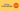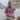# Infinity in JavaScript

Posted December 17, 2019Start discussion

`Infinity` in JavaScript is a special number with an interesting property: it’s bigger than any finite number.

Without knowing the properties of `Infinity` in advance, you might be surprised how infinite numbers perform in conditionals and arithmetical operations.

Let’s look at the properties of `Infinity` number in JavaScript, understand the use cases and be aware of common pitfalls.

## 1. The definition of Infinity

The ECMAScript standard describes `Infinity` as follows:

There are two other special values, called positive Infinity and negative Infinity. […] Note that these two infinite Number values are produced by the program expressions `+Infinity` (or simply `Infinity`) and `-Infinity`.

Which means that `Infinity`, as well as `-Infinity` (the number small than any finite number) are special values of type number:

``````typeof Infinity;  // => 'number'
typeof -Infinity; // => 'number'``````

`Infinity` is a property on the global object. For instance in a browser:

``window.Infinity; // => Infinity``

Note that `Number` function has 2 properties holding the infinite values as well:

``````Number.POSITIVE_INFINITY; // => Infinity
Number.NEGATIVE_INFINITY; // => -Infinity``````

## 2. The properties of Infinity

`Infinity` is bigger than any finite number.

Let’s see some examples:

``````Infinity > 100;                     // => true
Infinity > Number.MAX_SAFE_INTEGER; // => true
Infinity > Number.MAX_VALUE;        // => true``````

`Infinity` has interesting effects when used as an operand in arithmetical operations like addition, multiplication and division:

``````Infinity + 1;        // => Infinity
Infinity + Infinity; // => Infinity

Infinity * 2;        // => Infinity
Infinity * Infinity; // => Infinity

Infinity / 2;        // => Infinity``````

Some operations with `Infinity` result in finite numbers:

``10 / Infinity; // => 0``

Dividing a finite number by `0` results in `Infinity`:

``2 / 0; // => Infinity``

Making conceptually incorrect operations on infinite numbers results in `NaN`. For example, you can’t divide infinite numbers, and you cannot determine if an infinite number is odd or even:

``````Infinity / Infinity; // => NaN
Infinity % 2;        // => NaN``````

### 2.1 The negative infinity

`-Infinity` (the negative infinity) is smaller than any finite number.

Let’s compare `-Infinity` with some finite numbers:

``````-Infinity < 100;                      // => true
-Infinity < -Number.MAX_SAFE_INTEGER; // => true
-Infinity < -Number.MAX_VALUE;        // => true``````

At the same time, negative infinity is smaller than positive infinity:

``-Infinity < Infinity; // => true``

You might end up in negative infinity when using operands of different signs:

``````Infinity * -1; // => -Infinity
Infinity / -2; // => -Infinity
-2 / 0;        // => -Infinity``````

## 3. Checking for Infinity

Fortunately, an infinite value equals to an infinite value of the same sign:

``````Infinity === Infinity; // => true
-Infinity === -Infinity; // => true``````

Because of different signs `Infinity` doesn’t equal `-Infinity`:

``Infinity === -Infinity; // => false``

JavaScript has a special function `Number.isFinite(value)` that checks whether the provided value is finite:

``````Number.isFinite(Infinity);  // => false
Number.isFinite(-Infinity); // => false
Number.isFinite(999);       // => true``````

## 4. Infinity use cases

The infinity value is handy when you’d like to initialize computations involving comparisons of numbers.

For example, when searching for a minimum value in an array:

``````function findMin(array) {
let min = Infinity;  for (const item of array) {
min = Math.min(min, item);
}
return min;
}

findMin([5, 2, 1, 4]); // => 1``````

The `min` variable is initialized with `Infinity`. On first `for()` iteration the minimum value becomes the first item.

## 5. Pitfalls of Infinity

Most likely you won’t work directly with `Infinity` values so often. However, it worth knowing when the infinite values could appear.

### 5.1 Parsing numbers

Let’s say JavaScript uses an input (POST request, value from an input field, etc) to parse a number. In simple cases it would work fine:

``````// Parses the float number
parseFloat('10.5'); // => 10.5

// Indicates an invalid number
parseFloat('ZZZ'); // => NaN``````

Care must be taken because `'Infinity'` string is parsed by `parseFloat()` as an actual `Infinity` number:

``parseFloat('Infinity'); // => Infinity``

It should be a validation error when the user introduces the `'Infinity'` string into a numeric input field.

An alternative could be `parseInt()` to parse integers. It doesn’t recongize `'Infinity'` as an integer:

``````parseInt('10', 10); // => 10

parseInt('Infinity', 10); // => NaN``````

### 5.2 JSON serialization

`JSON.stringify()` serializes an `Infinity` number to `null`.

``````const worker = {
salary: Infinity
};

JSON.stringify(worker); // => '{ "salary": null }'``````

`salary` property is `Infinity`. But when stringified to JSON, `"salary"` becomes `null`.

### 5.3 Max number overflow

`Number.MAX_VALUE` is the biggest float number in JavaScript.

Trying to use a number even bigger than `Number.MAX_VALUE`, JavaScript transforms such number to `Infinity`:

``````2 * Number.MAX_VALUE; // => Infinity
Math.pow(10, 1000);   // => Infinity``````

### 5.4 Math functions

Some functions of `Math` namespace in JavaScript can return infinite numbers.

Here are a few examples:

``````const numbers = [1, 2];
const empty = [];

Math.max(...numbers); // => 2
Math.max(...empty);   // => -Infinity
Math.min(...numbers); // => 1
Math.min(...empty);   // => Infinity``````

`Math.max()` when invoked without arguments returns `-Infinity`, and `Math.min()` correspondingly `Infinity`. That could be a surprise if you try to determine the max or min of an empty array.

Here’s an interesting math discussion why that happens.

## 6. Key takeaway

`Infinity` in JavaScript represents the concept of an infinite number. Any finite number is smaller than `Infinity`, and any finite number is bigger `-Infinity`.

Comparing infinite values in JavaScript is easy: `Infinity === Infinity` is `true`. The special function `Number.isFinite()` determines if the supplied argument is a finite number.

You can initialize variables with `Infinite` when starting an algorithm that involves a comparison of numbers. A use case is finding the minimum of an array.

Care must be taken with `Infinity` when parsing numbers from inputs: `Number('Infinity')`, `parseFloat('Infinity')` return the actual `Infinity` number. When serialized with `JSON.stringify()`, the infinite number becomes `null`.

Hopefully, after reading my post you have a better idea of infinite numbers!

## Quality posts into your inbox

I regularly publish posts containing:

• Important JavaScript concepts explained in simple words
• Overview of new JavaScript features
• How to use TypeScript and typing
• Software design and good coding practices